Next: Pop-out and line Up: Long Range Connections Previous: Long Range Connections

## Stimulus Dependent Synchronization: a Mechanism for Binding

The cells are orientation selective and spatially organized according to a two-dimensional model orientation preference map [37,61]; see Fig. 10. In addition to the center-surround connectivity (discussed above), each cell has 10 long-range connections, chosen in the range between 25-50 lattice constants which connect it only with cells of similar orientation preference (difference in preferred orientation less than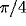) reproducing the pattern of long range clustered connections found in physiological studies [14,28,29]. In these simulations we assume that the long-range connections between cells with similar orientations are excitatory (but see the following section).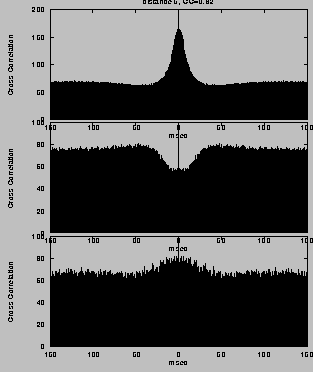Activity in neuronal array Cross-correlation functions for different separations

Figure 10: (click on the image to view a larger version) Activity in neuronal array. Shown is the array of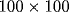cells where the gray-level of each pixel corresponds to the preferred orientation of the respective cell (lightest gray: horizontal, light gray: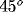, dark gray: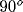, black: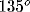). White pixels represent spiking cells. At this time, most of the activity is in regions with preferred orientation of about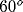.

External input is modeled independently for each cell as a Poisson process of excitatory voltage pulses of size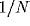, arriving at a mean rate which depends on the phase difference between the stimulus orientation and the cell's preferred orientation. The simulations were performed under two conditions:

1. A control, no-stimulus situation, in which the rate of the Poisson process received by each cell is the same (this could also be interpreted as spontaneous activity or as a noisy visual stimulus exciting all orientation cells alike).
2. A simulation with 2 stimuli of different orientations. All cells in the left half plane received a stimulus with a specific orientation, while cells in the right half plane received a stimulus with an orthogonal orientation.

In particular, we compare the strength of synchronization in the stimulus condition between cells that receive the same stimulus (both in the right half plane or both in the left half plane) versus cells which receive mutually orthogonal stimuli. Figure 10, left shows a snapshot of the activity in the 2-dimensional array, superimposed on the underlying orientation column structure.

For mutually excitatory pairs of cells, the cross-correlations show a `castle'  with a width of about 10-20 msec and centered at zero time offset (Fig. 5, top right). The cross-correlation function between model neurons at larger distance shows a weaker, slower ``hill'' (Fig. 5, bottom right). For mutually inhibitory cells the correlation function is characterized by a dip that rises to the coincidence level at longer times (Fig. 5, center right).

Figure 11: (click on the image to view a larger version) Correlation coefficient as a function of distance (in lattice constants) All curves are averaged over populations of neurons. (A) Homogeneous stimulation. Diamonds: correlation coefficient between cells of like orientation (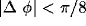), crosses: between cells of orthogonal orientation (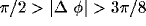;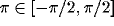). For distances larger than about 35 lattice constants, correlations between cells with common orientations (diamonds) become stronger than those between orthogonal orientations. (B) Differential stimulation. Diamonds: correlation coefficient between cells receiving stimulation with different orientation, crosses: correlation coefficient between cells receiving stimulation with the same orientation. Both curves in (B) are averaged over cells with all orientations. Note that for r between 30 and 45 (about the range of long-distance connections), pairs of cells receiving input with similar orientation are more strongly correlated than cells receiving input with orthogonal orientations, even if the members of the pair have with different preferred orientation.

We quantify the strength of synchronization by the contribution coefficient of the cross-correlation . This quantity measures the number of coincidences above chance level and is computed as the area of the cross-correlation above the asymptote divided by the total number of spikes. Both in the control case as well as in the simulation with oriented external input (Fig. 11), the contribution coefficient exhibits a spatial oscillation, with largest values at small distances (for neighboring cells), then becoming negative at about 15-30 lattice units (indicating anti-correlated activity), and then becoming positive again at the typical distances of the long range clustered connections (35-45 lattice constants).

In the control situation, the contribution coefficient at large distances is larger for similar vs. orthogonal orientations. For the 2-orientation stimuli, the contribution coefficient of cells with the same separation (30-45) is larger for cells belonging to the same half plane than for cells across the boundary between the two half s of the system that receive different stimuli (even if the later have the same orientation preference).

We have also computed the effect of stimulus-dependent synchronization on the basis of the LFP variables. We have computed the mean LFP cross-correlations between two recording sites, stimulated either by the same or by different gratings, within the same distance between each pair of sites. As shown in Figure 12, the correlation between cells receiving the same input is significantly stronger than that between cells that receive different inputs. One should notice that this effect is stimulus dependent and can not be explained by the simple fact that connected cells synchronize . In fact, the degree of correlation between two given cells depends their stimulation and is not only a function of the connectivity.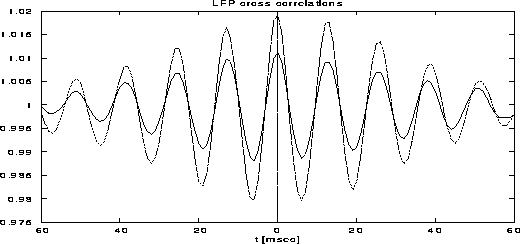Figure 12: Cross-correlation functions of local field potentials. The dotted curve is between populations of cells with the same preferred orientation and the full line is between different preferred orientations.

The mechanism responsible for stimulus dependent synchronization is the following:

1. Nearby cells are partially synchronized due to the short range excitatory connections, independent of their orientation preference. However cells whose preferred orientation equals that of their respective stimulus fire at a higher rate. Non-optimally oriented cells spike less, but when they do, they are in synchrony with their neighboring cells of optimal orientation preference.
2. Cells at larger distances excite each other only if they have the same orientation preference. Therefore, if two cells separated by a distance in the range of the clustered connections receive the same stimulus (even if they are not optimally tuned themselves), they indirectly synchronize via the intermediate synchronization with their optimally tuned neighboring cells.
3. For cells at similar distances that receive orthogonal stimuli, the synchronization link is less effective, since the optimally oriented cells in their vicinity have no direct connections.

Thus we find stimulus-dependent synchronization for cells in the range of the clustered connections. In accordance with recent experimental findings  and with other modeling work , we find that the degree of synchronization is higher when cells are excited with the same orientation. Synchronization is strong between nearby cells (independent of orientation preference) and there is a clear tendency for synchronization between cells with the same orientation preference separated by larger distances. We find that this result does not require models based on abstract phase oscillators with amplitude-dependent interactions, as in , but can be obtained in the more biologically plausible framework of populations of integrate-and-fire neurons.Next: Pop-out and line Up: Long Range Connections Previous: Long Range Connections